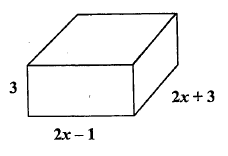MAT-HS.A-CED.01.e

 MAT-HS Targeted Standards(A) Concept: Algebra(CED) Domain: Creating ExpressionsCluster: Create equations that describe numbers or relationships MAT-HS.A-CED.01.e Create quadratic equations

• I can

• I can

• I can

• I can

Proficiency Scale

Score   Description Sample Activity

4.0

In addition to Score 3.0, the student demonstrates in-depth inferences and applications regarding more complex material that go beyond end of instruction expectations.
3.5 In addition to Score 3.0 performance, the student demonstrates in-depth inferences and applications regarding the more complex content with partial success.

3.0

(proficient)

The student can:
• create quadratic equations in one variable and use them to solve problems.
The student exhibits no major errors or omissions.

A square has an unknown side length of x.  A rectangle has a side length that is four feet longer than the square and a width that is two feet shorter than the square.  The areas of both the square and the rectangle are equal.  Find the side length of the square.

2.5 The student demonstrates no major errors or omissions regarding the simpler details and processes (Score 2.0 content) and partial knowledge of the more complex ideas and processes (Score 3.0 content).

2.0

(progressing)

There are no major errors or omissions regarding the simpler details and processes as the student can:

• recognize and recall specific terminology such as:
• equation
• create the equation; however, is unable to solve.

However, the student exhibits major errors or omissions regarding the more complex ideas and processes.

 What is the expression that represents the volume of the following prism?1.5 The student demonstrates partial knowledge of the simpler details and processes (Score 2.0 content) but exhibits major errors or omissions regarding the more complex ideas and procedures (Score 3.0 content).

1.0

(beginning)

With help, the student demonstrates a partial understanding of some of the simpler details and processes (Score 2.0 content) and some of the more complex ideas and processes (Score 3.0 content).
0.5 With help, the student demonstrates a partial understanding of some of the simpler details and processes (Score 2.0 content) but not the more complex ideas and processes (Score 3.0 content).
0.0 Even with help, the student demonstrates no understanding or skill.

Resources

 Web Vocab